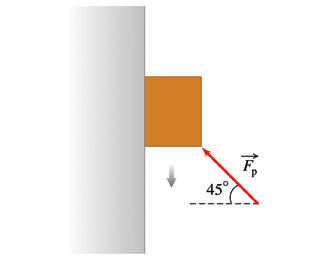# Problem: A box of mass 3.06 kg slides down a rough vertical wall. The gravitational force on the box is 30 N. When the box reaches a speed of 2.5 m/s, you start pushing on one edge of the box at a 45° angle (use degrees in your calculations throughout this problem) with a constant force of magnitude Fp = 23 N, as shown in (Figure 1). There is now a frictional force between the box and the wall of magnitude 13 N. How fast is the box sliding 2.8 s after you started pushing on it?Find the box's speed vf at 2.8 s after you first started pushing on it.

###### FREE Expert Solution
88% (173 ratings)###### Problem Details

A box of mass 3.06 kg slides down a rough vertical wall. The gravitational force on the box is 30 N. When the box reaches a speed of 2.5 m/s, you start pushing on one edge of the box at a 45° angle (use degrees in your calculations throughout this problem) with a constant force of magnitude Fp = 23 N, as shown in (Figure 1). There is now a frictional force between the box and the wall of magnitude 13 N. How fast is the box sliding 2.8 s after you started pushing on it?

Find the box's speed vf at 2.8 s after you first started pushing on it.